# How to count in a For or While Loop in PythonLast updated: Feb 19, 2023
5 min## #Count in a for loop in Python

Use the `enumerate()` function to count in a for loop.

The function takes an iterable and returns an object containing tuples, where the first element is the index, and the second is the item.

main.py
```Copied!```my_list = ['a', 'b', 'c']

# ✅ count in for loop

for index, item in enumerate(my_list):
print(index, item)

# 👇️
# 0 a
# 1 b
# 2 c
``````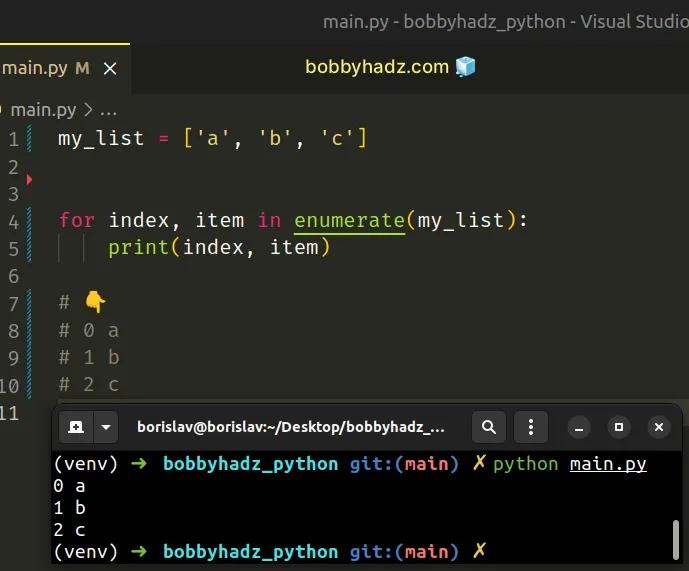The enumerate function takes an iterable and returns an enumerate object containing tuples where the first element is the index, and the second is the item.

We can directly unpack the index (or count) and the item in our `for` loop.

main.py
```Copied!```my_list = ['a', 'b', 'c']

for index, item in enumerate(my_list):
print(index, item)

# 👇️
# 0 a
# 1 b
# 2 c
``````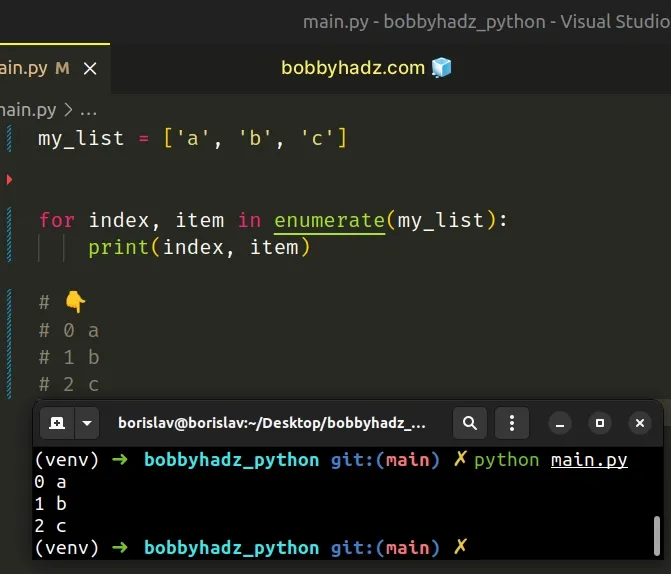The `enumerate` function takes an optional `start` argument, which defaults to `0`.

Note: if you need to count in a WHILE loop, click on the following subheading:

## #Count in a for loop starting from a different number

If you need to start the count from a different number, e.g. `1`, specify the `start` argument in the call to `enumerate()`.

main.py
```Copied!```my_list = ['a', 'b', 'c']

for count, item in enumerate(my_list, start=1):
print(count, item)

# 👇️
# 1 a
# 2 b
# 3 c
``````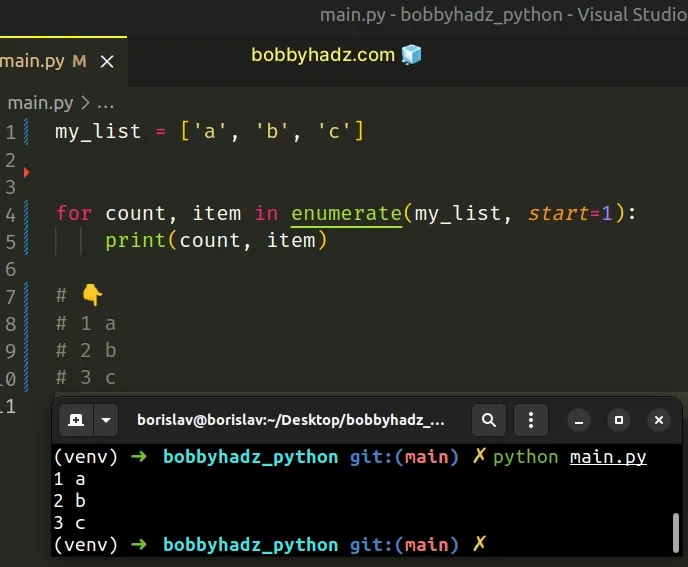The `count` variable has an initial value of `1` and then gets incremented by `1` on each iteration.

Alternatively, you can manually count in the `for` loop.

## #Counting in a for loop manually in Python

This is a three-step process:

1. Initialize a `count` variable and set it to a number.
2. Use a `for` loop to iterate over a sequence.
3. On each iteration, reassign the `count` variable to its current value plus N.
main.py
```Copied!```my_list = ['a', 'b', 'c']

count = 0

for item in my_list:
count += 1
print(count)

print(count)  # 👉️ 3
``````

We declared a `count` variable and initially set it to `0`.

On each iteration, we use the `+=` operator to reassign the variable to its current value plus N.

The following 2 lines of code achieve the same result:

• `count += 1`
• `count = count + 1`

Here is an example that uses the longer reassignment syntax.

main.py
```Copied!```my_list = ['a', 'b', 'c']

count = 0

for item in my_list:
count = count + 1
print(count)

print(count)  # 👉️ 3
``````

## #Counting in a for loop using range()

You can also use the `range()` class to count in a `for` loop.

main.py
```Copied!```a_list = ['bobby', 'hadz', 'com']

for index in range(len(a_list)):
# 0 bobby
# 2 com
print(index, a_list[index])
``````

The `range` class creates an iterator object of the specified length.

This approach is very similar to using `enumerate()`, however, the `enumerate` function is more convenient and direct.

## #Counting in a While loop in Python

To count in a `while` loop:

1. Declare a `count` variable and set it to `0`.
2. Use a `while` loop to iterate as long as `count` is less than N.
3. On each iteration, increment the value of the `count` variable by `1`.
main.py
```Copied!```count = 0

max_count = 5

while count < max_count:
count += 1
print(count)  # 👉️ 1 2 3 4 5
``````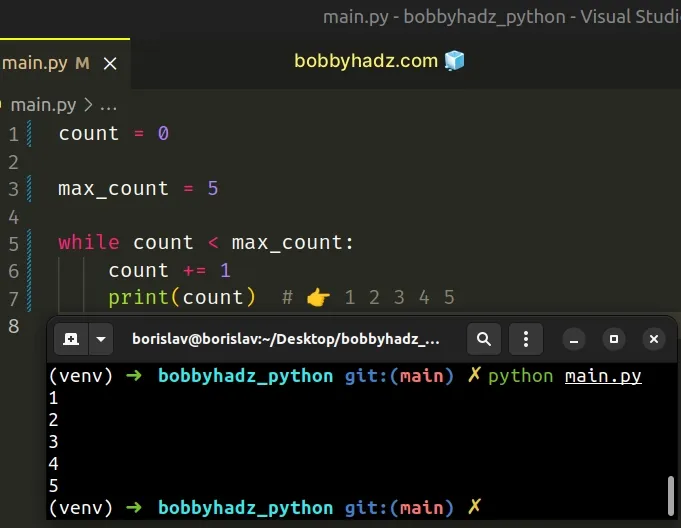We declared a `count` variable and set it to `0`.

The `max_count` variable is used in the condition of the `while` loop.

The `while` loop keeps iterating until the value of `count` is equal to or greater than the value of `max_count`.

On each iteration, we increment the value of the `count` variable by `1` to move towards the case where the condition is no longer met.

## #Counting how many times a while loop runs

You can also use this approach to count how many times a `while` loop runs.

main.py
```Copied!```count = 0

while len(my_list) > 0:
my_list.pop()

count += 1
print(count)  # 👉️ 1 2 3

print(count)  # 👉️ 3
``````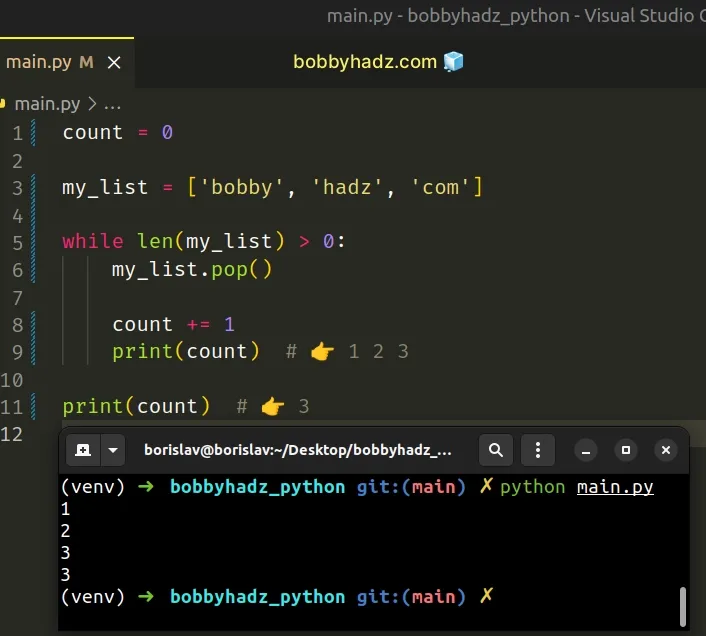On each iteration of the `while` loop, we increment the `count` variable and remove an item from a list.

The `while` loop keeps iterating and counting until the list is empty.

The `+=` operator is a shorthand for `count = count + 1`.

The following code sample achieves the same result using the more verbose syntax.

main.py
```Copied!```count = 0

while len(my_list) > 0:
my_list.pop()

count = count + 1
print(count)  # 👉️ 1 2 3

print(count)  # 👉️ 3
``````

If you don't have a condition and only need to iterate N times and keep track of the count, you can also use the `range()` class.

The range class is commonly used for looping a specific number of times in `for` loops and takes the following arguments:

NameDescription
`start`An integer representing the start of the range (defaults to `0`)
`stop`Go up to, but not including the provided integer
`step`Range will consist of every N numbers from `start` to `stop` (defaults to `1`)

If you only pass a single argument to the `range()` constructor, it is considered to be the value for the `stop` parameter.

main.py
```Copied!```for n in range(5):
print(n)

result = list(range(5))

# 👇️ [0, 1, 2, 3, 4]
print(result)
``````
The example shows that if the `start` argument is omitted, it defaults to `0` and if the `step` argument is omitted, it defaults to `1`.

If values for the `start` and `stop` parameters are provided, the `start` value is inclusive, whereas the `stop` value is exclusive.

main.py
```Copied!```result = list(range(1, 5))

# 👇️ [1, 2, 3, 4]
print(result)
``````

The `range()` class is more intuitive to use if you know how many times you need to iterate in advance.